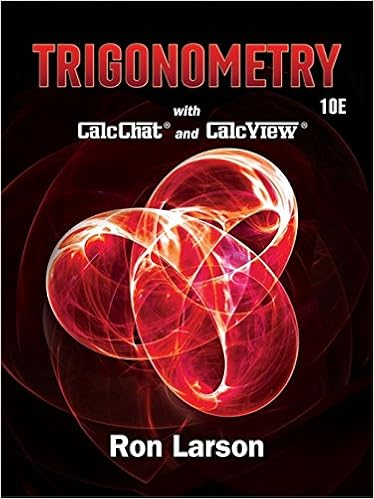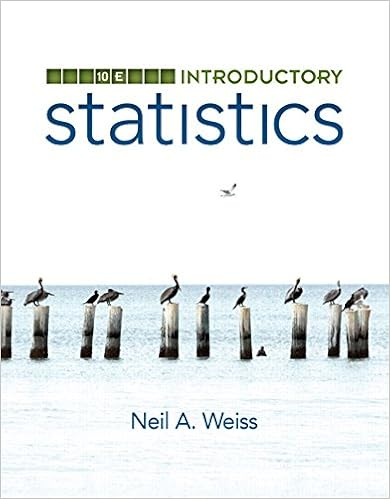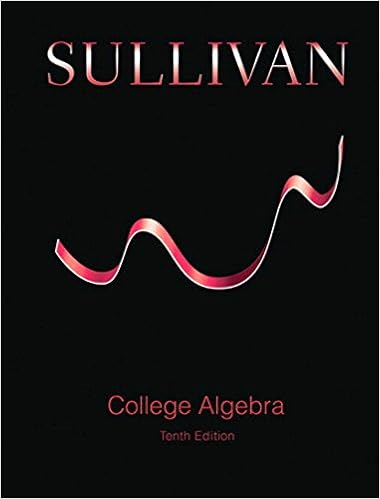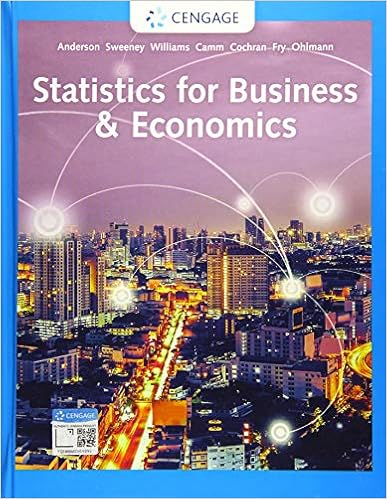# 1 construct the di ff erential equation for q t 2

• Notes
• 59
• 100% (2) 2 out of 2 people found this document helpful

This preview shows page 23 - 27 out of 59 pages.

(1) Construct the differential equation forQ(t).(2) Solve the differential equation.(3) Predict the long term quantity of the salt in the reservoir.
23
##### We have textbook solutions for you!The document you are viewing contains questions related to this textbook.
Chapter 6 / Exercise 8
Trigonometry
LarsonExpert VerifiedBrowse all Textbook Solutions
7.4 Exponential Growth and DecayIf the population growthdPdtis proportional to the population sizeP(t), thendPdt=kP.The solution isP(t) =P(0)ekt.k >0growth,k <0decay.Absolute growth rate = rate of change of population =dP/dt,Relative growth rate = percent change per unit time = (dP/dt)/P,Doubling time (D) of exponential growth = the time required for it to double:P(t) =P(0)2t/D.Half life (H) of exponential growth = the time required for it to be half:P(t) =P(0)12t/H.Newtons Law of Cooling states that the rate of change of the temperature of a coolingbody is proportional to the difference between its temperature T and the temperatureof its surrounding mediumTs. The model is:dTdt=-k(T-Ts),wherekis a constant.Example.A bacteria culture growth at a rate proportional to its size. After 2 hours thereare 40 bacteria and after 4 hours the count is 120. Find an expression for the populationafter t hours.
24
Note thatP(2) = 40 andP(4) = 120, we obtain40 =P(0)e2k,120 =P(0)e4k.These imply thatP(0) =403ande2k= 3,ork= ln 3/2.We thus haveP(t) =4033t/2=4033t=403e(ln 3/2)t.Example.The half-life of Sodium-24 is 15 hours. Suppose you have 100 grams of Sodium-24. How many grams remaining after 27 minutes (keep three decimals)?
Example.Use Newton’s Law of Cooling to determine the time of death of a healthy man.He died in his room some time before noon;At noon, his body temperature was found to be 70 degrees;His body cooled another 5 degrees in 1 hour after noon;The room temperature was a constant 60 degrees;Normal temperature of people’s body is 98.6 degree.
25
FromT(0) = 70 we get 70 = 60 +C,C= 10 andT(t) = 60 + 10e-kt.At 1:00pm, his body temperature is 70-5 = 65. HenceT(1) = 65.65 = 60 + 10e-k(1),5 = 10e-k,k= ln 2,T(t) = 60 + 10e-tln 2.Thus98.6 = 60 + 10e-tln 2,t=-ln 3.86/ln 2 =-1.95,which means 1 hour and 0.95(60) minutes before noon, or 1 hour and 57 minutes beforenoon. Or the time of death is 10:03AM.

Course Hero member to access this document

Course Hero member to access this document

End of preview. Want to read all 59 pages?

Course Hero member to access this document

Term
Winter
Professor
Kousha
Tags
dx, lim P, example
##### We have textbook solutions for you!
The document you are viewing contains questions related to this textbook.The document you are viewing contains questions related to this textbook.
Chapter 6 / Exercise 8
Trigonometry
LarsonExpert Verified
•••